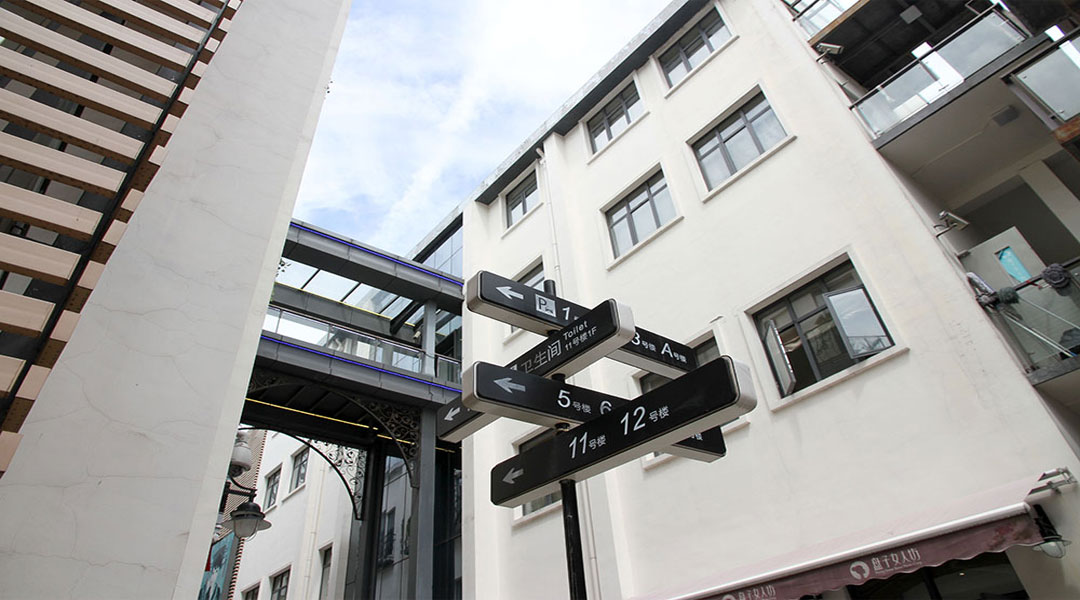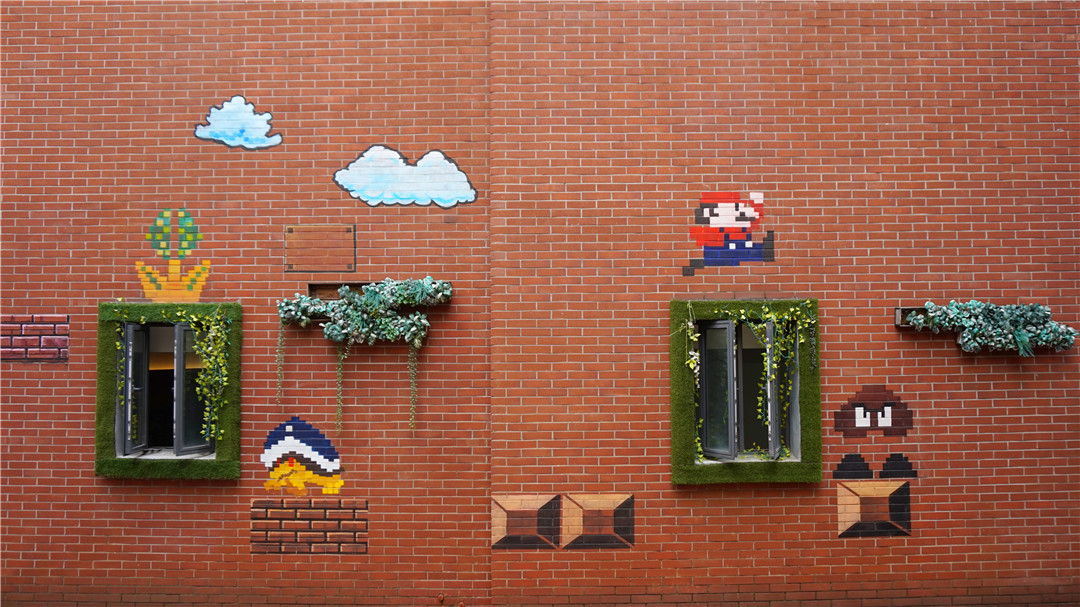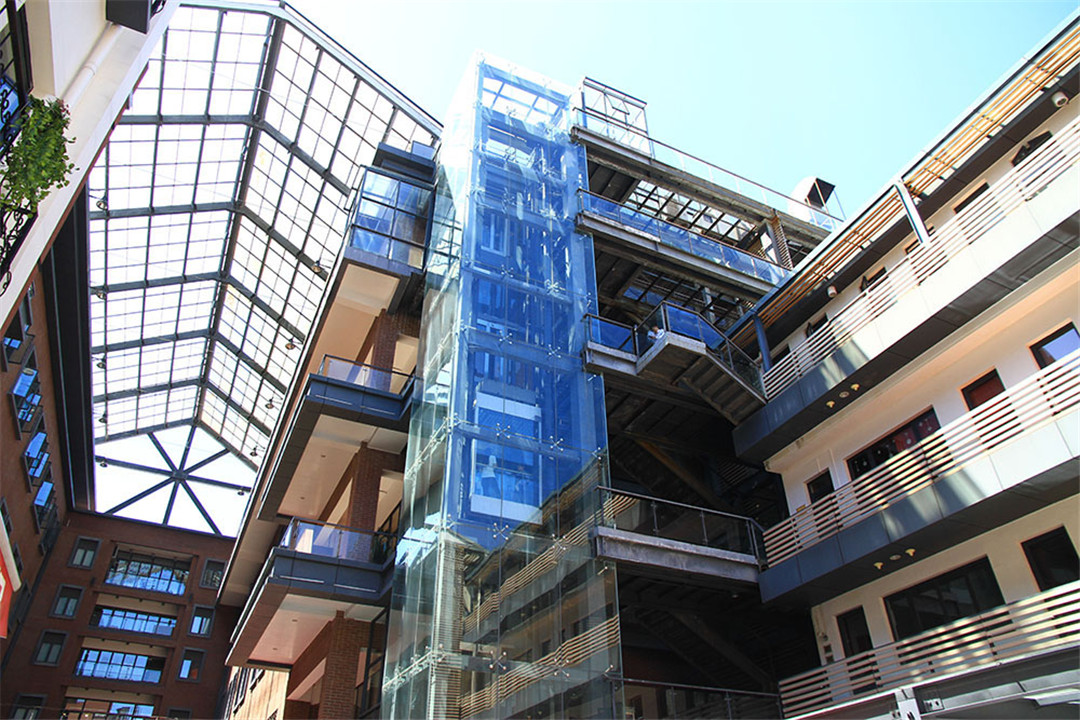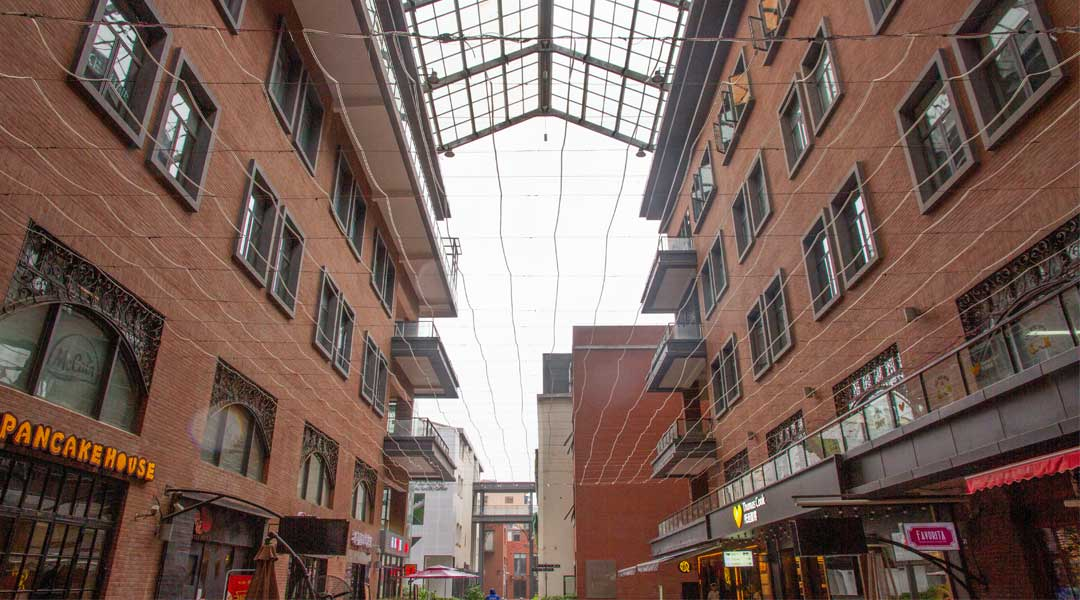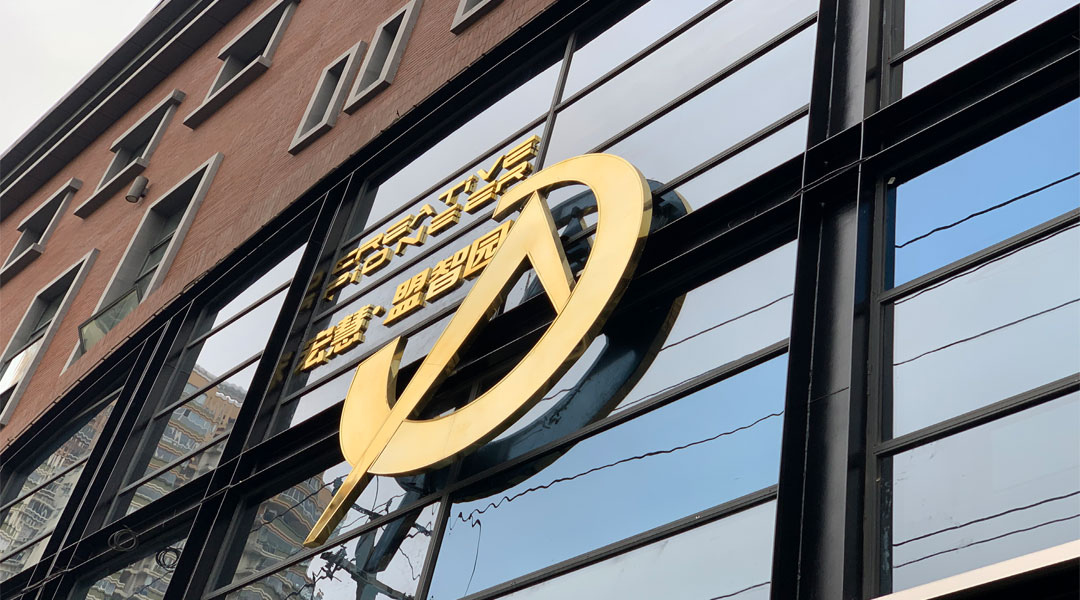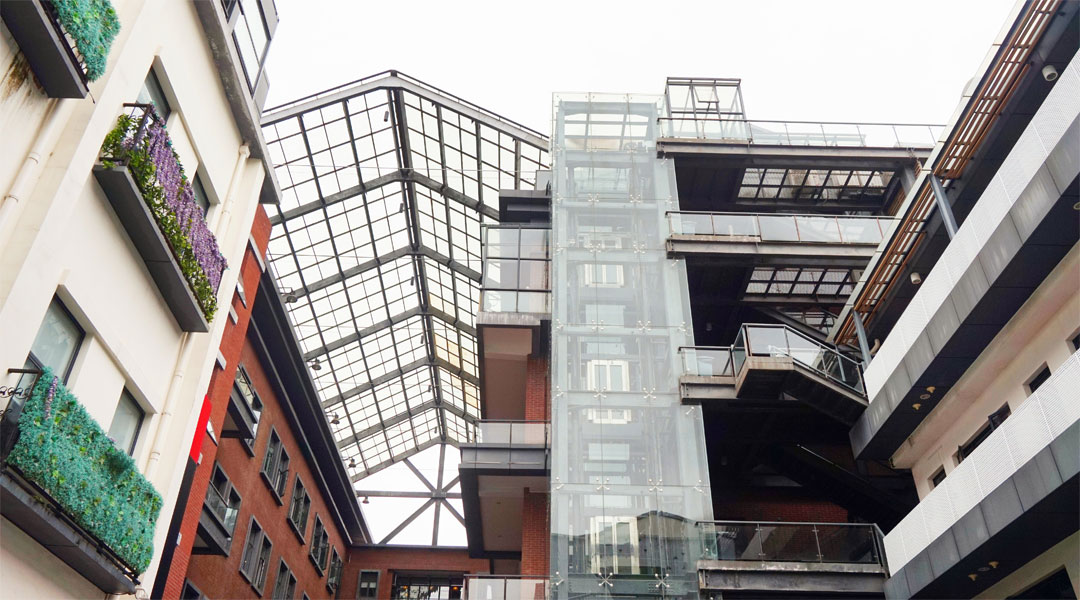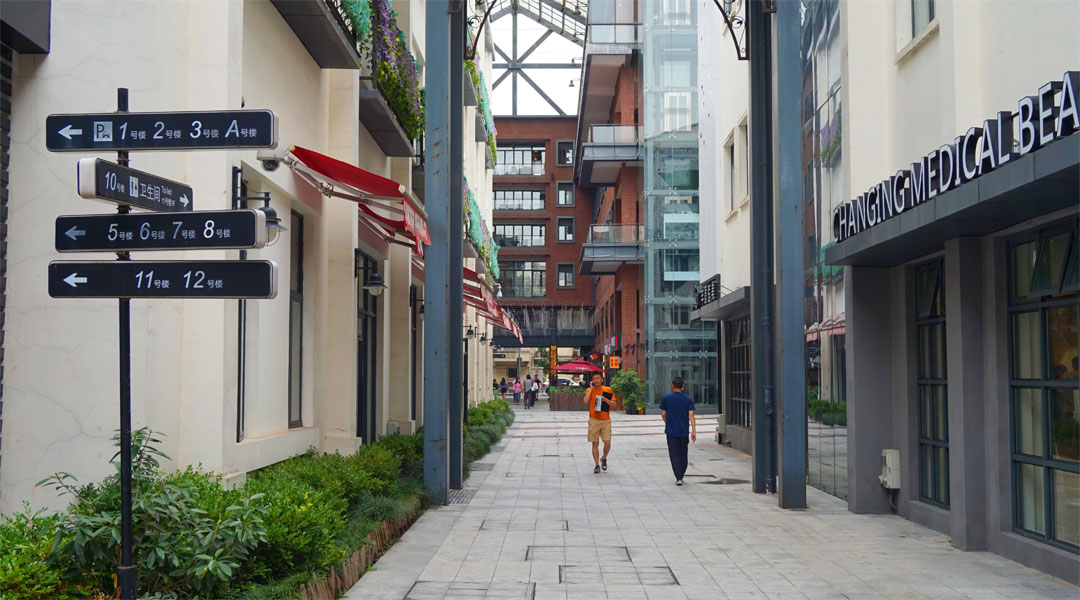{msg=success, data={columnId=96, navBreadcrumb=[{brother=[], entityTag=, id=null, name=项目介绍, type=false, url=/column/119/}, {brother=[], entityTag=, id=null, name=宏慧创意, type=false, url=/column/119/}, {brother=[], entityTag=, id=null, name=创意园区, type=false, url=/column/119/}, {brother=[], entityTag=, id=null, name=宏慧·盟智园, type=false, url=/column/96/}], list=[{columnId=96, name=宏慧·盟智园, linkUrl=/column/96/}, {columnId=104, name=宏慧·LAB 2354, linkUrl=/column/104/}, {columnId=98, name=宏慧·视界BOX, linkUrl=/column/98/}, {columnId=99, name=宏慧·音悦湾, linkUrl=/column/99/}, {columnId=100, name=宏慧·新汇园, linkUrl=/column/100/}, {columnId=126, name=宏慧·虎丘贰柒, linkUrl=/column/126/}, {columnId=102, name=新杨湾880, linkUrl=/column/102/}, {columnId=101, name=新业坊·源创, linkUrl=/column/101/}], homePage={brother=[], entityTag=, id=null, name=index_1, type=false, url=/}}, status=101}